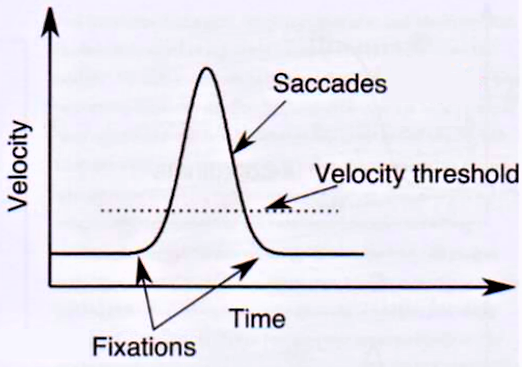# Fixation Filter

How does RealEye.io compute fixations?

## The Basics

Fixation is a series of gaze points that are very close in terms of time and space. To determine whether such a cluster of gazes is a fixation or not, some parameters need to be defined:

• minimum fixation duration,
• noise reduction,
• gaze velocity threshold.

In RealEye, we give you the following filters:There are all set by default (as shown above), but you can change them to suit your needs.

Noise reduction window is a time window (TOI - Time of Interest) that we use to denoise the raw data. Noise reduction using the moving median algorithm is a way to reduce the influence of noise on the actual results. Each point is replaced by a median of a number of points from the TOI (called noise reduction window).

Minimum fixation duration is a minimum time of gaze cluster (pause of the eye) that may be called a fixation. It typically ranges from 100 to 300 milliseconds.

Maximum fixation duration is the maximum time of a gaze cluster that may be called a fixation (it divides a bigger gaze cluster into several fixations).

Gaze velocity threshold - is a velocity "limit"; data below this limit is classified as fixation, while data that exceeds this velocity limit is a saccade. This filter may be set to a 0 value while having set a suitable noise reduction level.Maximum saccade duration - saccades are the eye movements between fixations (from one point of interest to another point of interest), so this filter allows determining when the eye movement is too long to be called a saccade.

NOTE 1:

Our fixation filter works for data with a sampling rate of over 20 Hz.

NOTE 2:

We consider gaze/fixation to be a part of an AOI if the fixation started in the AOI time range.

## Fixation Filter

RealEye uses an algorithm similar to the I-VT (Velocity-Threshold Identification) fixation filter.

Main rules for our algorithm:

• Gaze's max duration is 33 ms. The gaze ends at the raw gaze timestamp (time of eye-tracker prediction).
• Instead of angular velocity, we use velocity expressed as a percentage of the item size.
• We expand fixation time if the gap between gazes is not too big (not more than 50 ms).
• We don't calculate fixation if there aren't at least 2 gazes.
• The fixation coordinate point is the average of coordinates of all included gaze points.
• We calculate using numbers in IEEE 754 double-precision format (with decimal expansion to 14 decimal places).

Check out the data chart to see the influence of the filter settings.